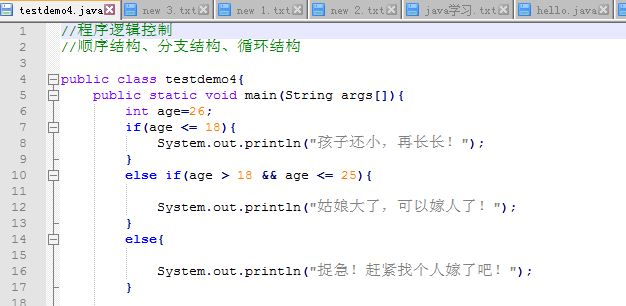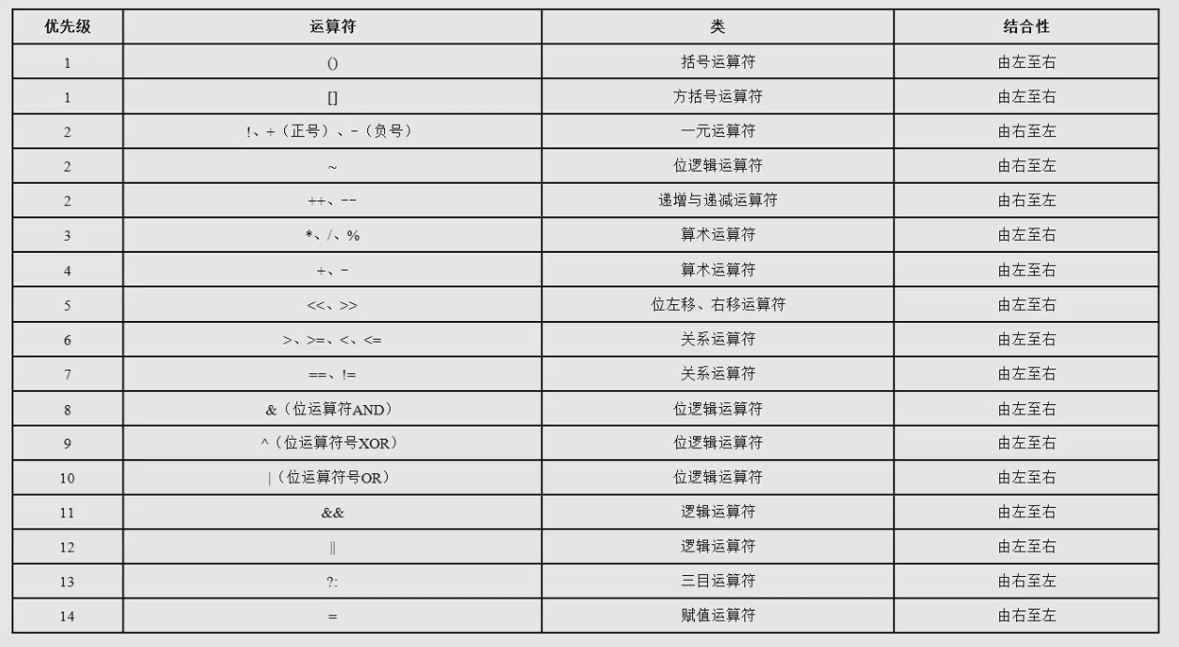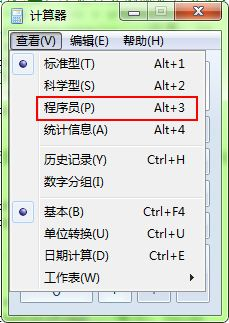## 微信小程序开发-轮播图

昨天下午刚开始学习小程序，各种注册申请流程，繁琐得很，好在花了一定时间完成了，然后就进入了开发。其实认识得很浅，慢慢学习吧，一步一步来。

## robot Framework（01）用户关键字的使用

（如图片看不清楚可点击图片显示原图）

## python robot Framework安装

https://www.ibm.com/developerworks/cn/opensource/os-cn-robot-framework/index.html

1、python 2.7下载安装（添加环境变量，重新打开cmd窗口）

## 库存系统性能优化感想-灵感很重要

最近两周做了一个库存系统的优化，其实工作量并不是很大，大概就用了几天的时间，但是对系统的影响是特别大的。

写这篇文章，主要是为了说明灵感的重要性，对于做性能优化这件事，就是一件再普通不过的事，并没有什么可说的。

## [java基础]5、java方法的定义和使用、方法重载

testdemo5.java

```/*
public static 方法返回值 方法名称([参数类型 变量,……]){
return 返回值;
}
*/
public class testdemo5{
public static void main(String args[]){
printTest();
int r = plus(10,20);
System.out.println(r);
r = plus(10,20,30);
System.out.println(r);
System.out.println(plus(10.1,20));
System.out.println(OneToN(50));
}
//方法定义：无返回值的方法
public static void printTest(){
System.out.println("test function!");
}
//方法定义：有返回值的方法
public static int plus(int a,int b){
return a + b;
}
//方法重载：方法名相同，参数的类型或个数不同，一般重载方法返回值类型相同
//3个参数，与2个参数的同名方法重载
public static int plus(int a,int b,int c){
return a + b + c;
}
public static double plus(double a,double b){
return a + b;
}
//方法的递归调用：自己调用自己，往往都有以下特点：
//方法必须有一个结束递归调用条件
//每次递归调用后都会有不同的变化
//计算1到n的累加总和
public static int OneToN(int n)
{
if(n <= 0) return 0;
else if(n == 1) return 1;
else return n + OneToN(n - 1);
}
//所有的while循环可以用递归代替，但用哪种取决于具体需求，哪种开发起来方便就用哪种
}```

## [java基础]4、java程序逻辑控制语句testdemo4.java

```public class testdemo4{
public static void main(String args[]){
int age=26;
if(age <= 18){
System.out.println("孩子还小，再长长！");
}
else if(age > 18 && age <= 25){
System.out.println("姑娘大了，可以嫁人了！");
}
else{
System.out.println("捉急！赶紧找个人嫁了吧！");
}
//分支结构
int day = 5;
switch(day){
case 1 :
{
System.out.println("一号");
}
break;
case 2 :
{
System.out.println("二号");
}
break;
default ://除上面情况外的其他情况
{
System.out.println("其他");
}
break;
}
//循环结构
int num = 1;
int result = 0;
//while循环（还有do...while，一般很少用）
while(num <= 100){
result += num;
num++;
}
System.out.println(result);
result = 0;
//for循环
for(num = 1;num<=100;num++)
{
result += num;
}
System.out.println(result);
//循环控制语句：continue（跳过当前循环）,break（退出整个循环）
lb: for(int x = 0; x < 5; x ++)
for(int y = 0;y < 3;y ++){
if(x > 2) continue lb;//回到执行x循环
System.out.println("x = " + x + ", y = "+y);
}
//循环嵌套
//打印乘法表
for(int x = 1; x <= 9; x ++){
for(int y = 1;y <= x;y ++){
System.out.print(x + "*" + y +"=" + x * y + "\t");
}
System.out.println();
}
int line = 6;
for(int x = 0; x < line; x ++){
for(int y = 0;y < line - x;y ++){
System.out.print(" ");
}
for(int y = 0;y < x;y ++){
System.out.print("* ");
}
System.out.println();
}
}
}```

## [java基础]3、java运算符windows二进制转换器：## [java基础]2、java中基本概念数据类型

CLASSPATH：

SET CLASSPATH=d:\mydemo

SET CLASSPATH=.;d:\mydemo

//也可以同样以设置环境变量的方式自动生效

//  单行注释

/*  多行注释 */

/** 文档注释 */